## General Equation For the Radius of Curvature of a Curve

If a curve is defined parametrically as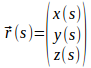where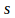is the length along the curve from some point, then the radius of curvature of the curve is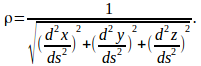We can prove this using the Frenet formula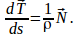Since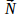is a unit vector,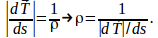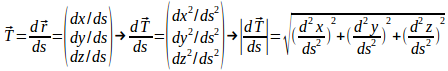Hence